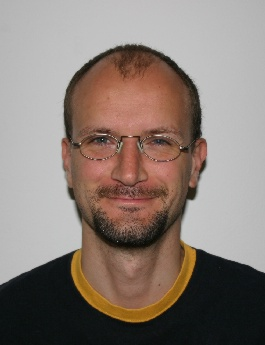Peter Mayr### Peter Mayr

I am working at the Department of Algebra at the University of Linz. Since 1997 I have been involved in the SONATA project, a GAP-package for the computation with near-rings.
From October 2006 to September 2007 I was visiting the Department of Mathematics at the University of Colorado at Boulder supported by the Austrian science fund (FWF) via an Erwin-Schrödinger-Grant. The topic of my research was clones of polynomial and term functions on groups and Mal'cev algebras. Here is a detailed description and here is one result.

### Teaching

Universität Linz:
FH Hagenberg:
• Übung Mathematik 3, Studiengang CMS (Winter 08/09) .
• Übung Sicherheit in Mobilsystemen, Studiengang MC (Winter 07/08).
Something completely different:

### Research

My current research interests are group theory, universal algebra, and their connections:
• clones (polynomial, term functions on algebraic structures, compatibility, interpolation, completeness); some typical results can be found on this poster.
• varieties (expanded groups, Malcev terms, equations in algebraic structures, finiteness conditions)
• actions of groups (infinite sharply 2-transitive permutation groups, Frobenius groups, representation theory)
Some questions for which I would like to have an answer:
• Is there an infinite sharply 2-transitive permutation group with point stabilizer of finite exponent?
• Let A be a subgroup of order pq (p,q not necessarily distinct primes) of the point stabilizer of an infinite sharply 2-transitive permutation group. Is A cyclic?
• (Mazurov, Kourovka Notebook 14.58) Let A be a group of prime exponent that acts Frobenius (ie, fixed-point-free, regular) on a vector space. Is A cyclic?
• Determine the finite subgroups of the multiplicative groups of near-fields.
• Is the transposition on a general linear group over some infinite field (reals, complex numbers,...) a polynomial function?

### Publications

Here you can find my papers.
For my presentations you can also look at the university's database for research documentation, FODOK.

### Contact

Visit me at my office at Linz University, room KG416.
Send me an e-mail: peter.mayr@jku.at
Try to skype me.
My phone number is ++43 732 2468 9143.
Our department has a fax with the number ++43 732 2468 8499.
My postal address: Department of Algebra, Johannes Kepler Universität Linz, Altenberger Strasse 69, 4040 Linz, Austria, Europe.